# 2nd order CR filter Design tools

This page is a web calculator 2nd order CR filter from combinations of two CR 1st order filters. Use this utility to calculate the Transfer Function for filters at a given values of R and C. The response of the filter is displayed on graphs, showing Bode diagram, Nyquist diagram, Impulse response and Step response.

## Calculate the transfer function for 2nd order CR low-pass filter with R and C values

 Vi(s)→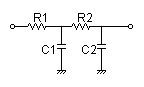→Vo(s)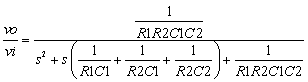R1=Ω C1=F R2=Ω C2=F
p:pico, n:nano, u:micro, k:kilo, M:mega

### Frequency analysis

Bode diagram
Phase  Group delay
Nyquist diagram
Pole, zero
Phase margin
Oscillation analysis
Analysis on frequency range:
f1=∼f2=[Hz] (optional)

### Transient analysis

Step response
Impulse response
Overshoot
Final value of the step response
Analysis on time range:
0∼[sec] (optional)

## Calculate the transfer function for 2nd order CR high-pass filter with R and C values

 Vi(s)→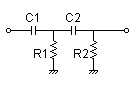→Vo(s)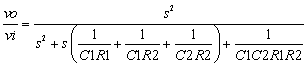R1=Ω C1=F R2=Ω C2=F
p:pico, n:nano, u:micro, k:kilo, M:mega

### Frequency analysis

Bode diagram
Phase  Group delay
Nyquist diagram
Pole, zero
Phase margin
Oscillation analysis
Analysis on frequency range:
f1=∼f2=[Hz] (optional)

### Transient analysis

Step response
Impulse response
Overshoot
Final value of the step response
Analysis on time range:
0∼[sec] (optional)

## Calculate the transfer function for 2nd order CR band-pass filter with R and C values

 Vi(s)→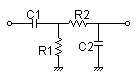→Vo(s)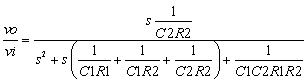R1=Ω C1=F R2=Ω C2=F
p:pico, n:nano, u:micro, k:kilo, M:mega

### Frequency analysis

Bode diagram
Phase  Group delay
Nyquist diagram
Pole, zero
Phase margin
Oscillation analysis
Analysis on frequency range:
f1=∼f2=[Hz] (optional)

### Transient analysis

Step response
Impulse response
Overshoot
Final value of the step response
Analysis on time range:
0∼[sec] (optional)